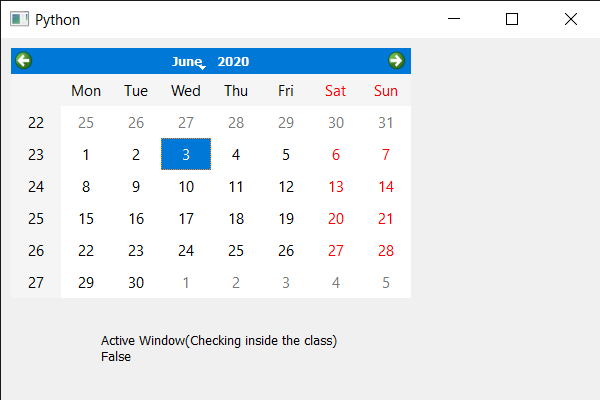# PyQt5 QCalendarWidget – Checking if it is active window or not

• Last Updated : 08 Jul, 2021

In this article, we will see how we can check if the QCalendarWidget is an active window. An active window is a visible top-level window that has the keyboard input focus. Making it an active window will set the top-level widget containing this calendar to be the active window. We can make it an active window with the help of activateWindow method.
Note: This method should be called outside the main window class else it will always return false

In order to do this we will use isActivateWindow method with the QCalendarWidget object.
Syntax : calendar.isActivateWindow()
Argument : It takes no argument
Action Performed : It return bool

Below is the implementation

## Python3

 `# importing libraries``from` `PyQt5.QtWidgets ``import` `*``from` `PyQt5 ``import` `QtCore, QtGui``from` `PyQt5.QtGui ``import` `*``from` `PyQt5.QtCore ``import` `*``import` `sys`  `class` `Window(QMainWindow):` `    ``def` `__init__(``self``):``        ``super``().__init__()` `        ``# setting title``        ``self``.setWindowTitle(``"Python "``)` `        ``# setting geometry``        ``self``.setGeometry(``100``, ``100``, ``600``, ``400``)` `        ``# calling method``        ``self``.UiComponents()` `        ``# showing all the widgets``        ``self``.show()` `    ``# method for components``    ``def` `UiComponents(``self``):` `        ``# creating a QCalendarWidget object``        ``self``.calendar ``=` `QCalendarWidget(``self``)` `        ``# setting geometry to the calendar``        ``self``.calendar.setGeometry(``10``, ``10``, ``400``, ``250``)` `        ``# setting name``        ``self``.calendar.setAccessibleName(``"Geek Calendar"``)` `        ``# making it an active window``        ``self``.calendar.activateWindow()`  `        ``# creating a label``        ``label ``=` `QLabel(``self``)` `        ``# setting geometry to the label``        ``label.setGeometry(``100``, ``280``, ``250``, ``60``)` `        ``# making label multi line``        ``label.setWordWrap(``True``)` `        ``# checking active window``        ``value ``=` `self``.calendar.isActiveWindow()` `        ``# setting text to the label``        ``label.setText(``"Active Window(Checking inside the class) "` `+` `str``(value))`  `# create pyqt5 app``App ``=` `QApplication(sys.argv)` `# create the instance of our Window``window ``=` `Window()` `# checking if active window``# outside the class``check ``=` `window.calendar.isActiveWindow()``print``(``"Active window : "` `+` `str``(check))` `# start the app``sys.exit(App.``exec``())`

Output :

`Active window : True`My Personal Notes arrow_drop_up